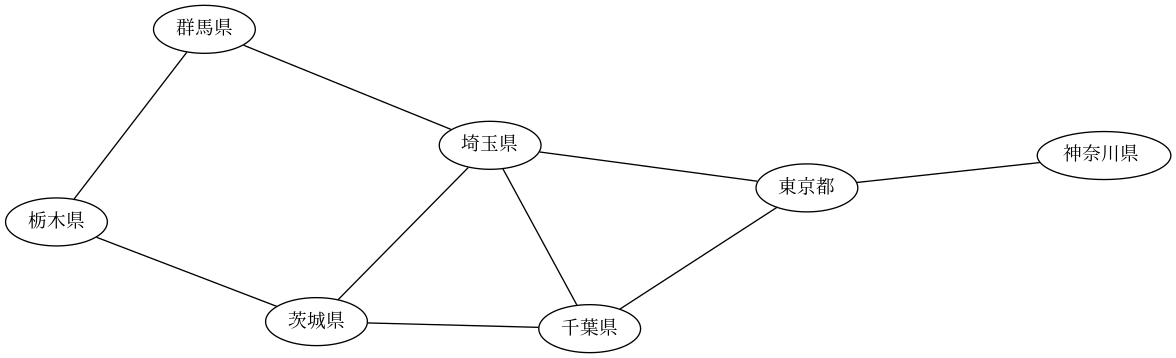# Expected value of X and 1/X

Yesterday I blogged about an exercise in the book The Cauchy-Schwarz Master Class. This post is about another exercise from that book, exercise 5.8, which is to prove Kantorovich’s inequality.

Assumeandfor non-negative numbers pi.

Thenwhereis the arithmetic mean of m and M andis the geometric mean of m and M.

In words, the weighted average of the x‘s times the weighted average of their reciprocals is bounded by the square of the ratio of the arithmetic and geometric means of the x‘s.

## Probability interpretation

I did a quick search on Kantorovich’s inequality, and apparently it first came up in linear programming, Kantorovich’s area of focus. But when I see it, I immediately think expectations of random variables. Maybe Kantorovich was also thinking about random variables, in the context of linear programming.

The left side of Kantorovich’s inequality is the expected value of a discrete random variable X and the expected value of 1/X.

To put it another way, it’s a relationship between E[1/X] and 1/E[X],which I imagine is how it is used in practice.

I don’t recall seeing this inequality used, but it could have gone by in a blur and I didn’t pay attention. But now that I’ve thought about it, I’m more likely to notice if I see it again.

## Python example

Here’s a little Python code to play with Kantorovich’s inequality, assuming the random values are uniformly distributed on [0, 1].

    from numpy import random

x = random.random(6)
m = min(x)
M = max(x)
am = 0.5*(m+M)
gm = (m*M)**0.5
prod = x.mean() * (1/x).mean()
bound = (am/gm)**2
print(prod, bound)


This returned 1.2021 for the product and 1.3717 for the bound.

If we put the code above inside a loop we can plot the product and its bound to get an idea how tight the bound is typically. (The bound is perfectly tight if all the x’s are equal.) Here’s what we get.All the dots are above the dotted line, so we haven’t found an exception to our inequality.

(I didn’t think that Kantorovich had made a mistake. If he had, someone would have noticed by now. But it’s worth testing a theorem you know to be true, in order to test that your understanding of the theorem is correct.)